International
Tables for
Crystallography
Volume D
Physical properties of crystals
Edited by A. Authier

International Tables for Crystallography (2006). Vol. D, ch. 1.1, pp. 17-20

## Section 1.1.4.8. Reduction of the components of a tensor of rank 3

A. Authiera*

aInstitut de Minéralogie et de la Physique des Milieux Condensés, Bâtiment 7, 140 rue de Lourmel, 75015 Paris, France
Correspondence e-mail: aauthier@wanadoo.fr

| top | pdf |

| top | pdf |

#### 1.1.4.8.1.1. Group| top | pdf |

All the components are independent. Their number is equal to 27. They are usually represented as a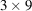matrix which can be subdivided into threesubmatrices: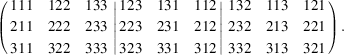#### 1.1.4.8.1.2. Group| top | pdf |

All the components are equal to zero.

| top | pdf |

#### 1.1.4.8.2.1. Group| top | pdf |

Choosing the twofold axis parallel to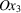and applying the direct inspection method, one findsThere are 13 independent components. If the twofold axis is parallel to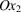, one finds#### 1.1.4.8.2.2. Group m

| top | pdf |

One obtains the matrix representing the operator m by multiplying by −1 the coefficients of the matrix representing a twofold axis. The result of the reduction will then be exactly complementary: the components of the tensor which include an odd number of 3's are now equal to zero. One writes the result as follows:There are 14 independent components. If the mirror axis is normal to, one finds#### 1.1.4.8.2.3. Group| top | pdf |

All the components are equal to zero.

| top | pdf |

#### 1.1.4.8.3.1. Group| top | pdf |

There are three orthonormal twofold axes. The reduction is obtained by combining the results associated with two twofold axes, parallel toand, respectively.There are 6 independent components.

#### 1.1.4.8.3.2. Group| top | pdf |

The reduction is obtained by combining the results associated with a twofold axis parallel toand with a mirror normal to:There are 7 independent components.

#### 1.1.4.8.3.3. Group| top | pdf |

All the components are equal to zero.

| top | pdf |

#### 1.1.4.8.4.1. Group| top | pdf |

The threefold axis is parallel to. The matrix method should be used here. One findsThere are 9 independent components.

#### 1.1.4.8.4.2. Groupwith a twofold axis parallel to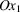| top | pdf |There are 4 independent components.

#### 1.1.4.8.4.3. Groupwith a mirror normal to| top | pdf |There are 4 independent components.

#### 1.1.4.8.4.4. Groupsand| top | pdf |

All the components are equal to zero.

| top | pdf |

#### 1.1.4.8.5.1. Group| top | pdf |

The method of direct inspection can be applied for a fourfold axis. One findsThere are 7 independent components.

#### 1.1.4.8.5.2. Group| top | pdf |

One combines the reductions for groups 4 and 222:There are 3 independent components.

#### 1.1.4.8.5.3. Group| top | pdf |

One combines the reductions for groups 4 and 2m:There are 4 independent components.

#### 1.1.4.8.5.4. Group| top | pdf |

All the components are equal to zero.

#### 1.1.4.8.5.5. Group| top | pdf |

The matrix corresponding to axisisand the form of thematrix isThere are 6 independent components.

#### 1.1.4.8.5.6. Group| top | pdf |

One combines either the reductions for groupsand 222, or the reductions for groupsand 2mm.

 (i) Twofold axis parallel to:There are 6 independent components. (ii) Mirror perpendicular to(the twofold axis is at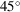)The number of independent components is of course the same, 6.

#### 1.1.4.8.5.7. Group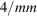| top | pdf |

All the components are equal to zero.

| top | pdf |

#### 1.1.4.8.6.1. Groups,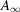,,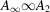,and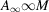| top | pdf |

It was shown in Section 1.1.4.6.2.3that, in the case of tensors of rank 3, the reduction is the same for axes of order 4, 6 or higher. The reduction will then be the same as for the tetragonal system.

#### 1.1.4.8.6.2. Group| top | pdf |

One combines the reductions for the groups corresponding to a threefold axis parallel toand to a mirror perpendicular to:There are 2 independent components.

#### 1.1.4.8.6.3. Group| top | pdf |

One combines the reductions for groups 6 and 2mm:There is 1 independent component.

#### 1.1.4.8.6.4. Groups,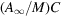,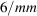and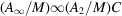| top | pdf |

All the components are equal to zero.

| top | pdf |

#### 1.1.4.8.7.1. Group| top | pdf |

One combines the reductions corresponding to a twofold axis parallel toand to a threefold axis parallel to :There are 2 independent components.

#### 1.1.4.8.7.2. Groupsand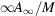| top | pdf |

One combines the reductions corresponding to groups 422 and 23:There is 1 independent component.

#### 1.1.4.8.7.3. Group| top | pdf |

One combines the reductions corresponding to groupsand 23:There is 1 independent component.

#### 1.1.4.8.7.4. Groups,and| top | pdf |

All the components are equal to zero.